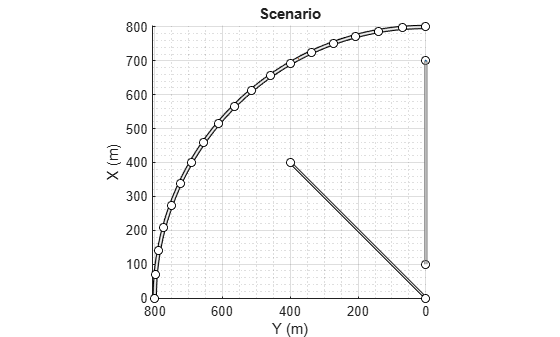Documentation

# actorPoses

Positions, velocities, and orientations of actors in driving scenario

## Syntax

``poses = actorPoses(scenario)``

## Description

example

````poses = actorPoses(scenario)` returns the current poses (positions, velocities, and orientations) for all actors in the driving scenario, `scenario`. Actors include `Actor` and `Vehicle` objects, which you can create using the `actor` and `vehicle` functions, respectively. Actor poses are in scenario coordinates.```

## Examples

collapse all

Create a driving scenario containing a curved road, two straight roads, and two actors: a car and a bicycle. Both actors move along the road for 60 seconds.

Create the driving scenario object.

`scenario = drivingScenario('SampleTime',0.1','StopTime',60);`

Create the curved road using road center points following the arc of a circle with an 800-meter radius. The arc starts at 0°, ends at 90°, and is sampled at 5° increments.

```angs = [0:5:90]'; R = 800; roadcenters = R*[cosd(angs) sind(angs) zeros(size(angs))]; roadwidth = 10; road(scenario,roadcenters,roadwidth);```

```roadcenters = [700 0 0; 100 0 0]; road(scenario,roadcenters) roadcenters = [400 400 0; 0 0 0]; road(scenario,roadcenters)```

`rbdry = roadBoundaries(scenario);`

Add a car and a bicycle to the scenario. Position the car at the beginning of the first straight road.

`car = vehicle(scenario,'Position',[700 0 0],'Length',3,'Width',2,'Height',1.6);`

Position the bicycle farther down the road.

`bicycle = actor(scenario,'Position',[706 376 0]','Length',2,'Width',0.45,'Height',1.5);`

Plot the scenario.

```plot(scenario,'Centerline','on','RoadCenters','on'); title('Scenario');```Display the actor poses and profiles.

`poses = actorPoses(scenario)`
```poses=2×7 struct ActorID Position Velocity Roll Pitch Yaw AngularVelocity ```
`profiles = actorProfiles(scenario)`
```profiles=2×9 struct ActorID ClassID Length Width Height OriginOffset RCSPattern RCSAzimuthAngles RCSElevationAngles ```

Create a driving scenario containing three vehicles. Find the target poses of two of the vehicles as viewed by the third vehicle. Target poses are returned in the ego-centric coordinate system of the third vehicle.

Create a driving scenario.

`scenario = drivingScenario;`

Create the target actors.

```actor(scenario,'Position',[10 20 30], ... 'Velocity',[12 113 14], ... 'Yaw',54, ... 'Pitch',25, ... 'Roll',22, ... 'AngularVelocity',[24 42 27]); actor(scenario,'Position',[17 22 12], ... 'Velocity',[19 13 15], ... 'Yaw',45, ... 'Pitch',52, ... 'Roll',2, ... 'AngularVelocity',[42 24 29]);```

```ego = actor(scenario,'Position',[1 2 3], ... 'Velocity',[1.2 1.3 1.4], ... 'Yaw',4, ... 'Pitch',5, ... 'Roll',2, ... 'AngularVelocity',[4 2 7]);```

Use `actorPoses` to return the poses of all the actors. Pose properties (position, velocity, and orientation) are in scenario coordinates.

`allposes = actorPoses(scenario);`

Use `driving.scenario.targetsToEgo` to convert only the target poses to the ego-centric coordinates of the ego actor. Examine the pose of the first actor.

```targetposes1 = driving.scenario.targetsToEgo(allposes(1:2),ego); disp(targetposes1(1))```
``` ActorID: 1 Position: [7.8415 18.2876 27.1675] Velocity: [18.6826 112.0403 9.2960] Roll: 16.4327 Pitch: 23.2186 Yaw: 47.8114 AngularVelocity: [-3.3744 47.3021 18.2569] ```

Alternatively, use `targetPoses` to obtain all non-ego actor poses in ego vehicle coordinates. Compare these poses to the previously calculated poses.

```targetposes2 = targetPoses(ego); disp(targetposes2(1))```
``` ActorID: 1 ClassID: 0 Position: [7.8415 18.2876 27.1675] Velocity: [18.6826 112.0403 9.2960] Roll: 16.4327 Pitch: 23.2186 Yaw: 47.8114 AngularVelocity: [-3.3744 47.3021 18.2569] ```

## Input Arguments

collapse all

Driving scenario, specified as a `drivingScenario` object.

## Output Arguments

collapse all

Actor poses, in scenario coordinates, returned as a structure or an array of structures. Poses are the positions, velocities, and orientations of actors.

Each structure in `poses` has these fields.

FieldDescription
`ActorID`

Scenario-defined actor identifier, specified as a positive integer.

`Position`

Position of actor, specified as an [x y z] real-valued vector. Units are in meters.

`Velocity`

Velocity (v) of actor in the x-, y-, and z-directions, specified as a [vx vy vz] real-valued vector. Units are in meters per second.

`Roll`

Roll angle of actor, specified as a real scalar. Units are in degrees.

`Pitch`

Pitch angle of actor, specified as a real scalar. Units are in degrees.

`Yaw`

Yaw angle of actor, specified as a real scalar. Units are in degrees.

`AngularVelocity`

Angular velocity (ω) of actor in the x-, y-, and z-directions, specified as an [ωx ωy ωz] real-valued vector. Units are in degrees per second.

For full definitions of these structure fields, see the `actor` and `vehicle` functions.